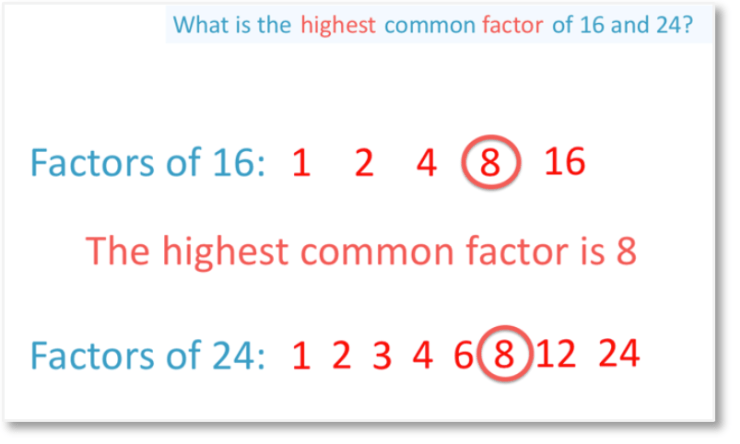# LCM & HCF and Perfect Squares

Welcome to Class !!

In today’s Mathematics class, We will be discussing LCM, HCF and Perfect Squares. We hope you enjoy the class!Highest Common Factors

A highest common factor is the greatest number which will divide exactly into two or more numbers. For example, 4 is the highest common factor (HCF) of 20 & 24.

i.e. 20 = 1, 2, (4), 5, 10, 20

24= 1, 2, 3, (4), 6, 8, 12, 24

Example:

Find the H.C.F of 24 & 78

Method 1

Express each number as a product of its prime factors

Workings

2       |   24                    2       |   78

2       |   12                    2       |   36

2       |  6                      2        |  18

3       |   3                      3       |   9

3       |  3

24=23x3

78 =(23 x 3) x 3

The H.C.F. is the product of the common prime factors.

HCF=23x3

=8×3=24

Method II

24=2x2x2x3

78=2x2x2x3x3

Common factor=2x2x2x3

HCF=24#### LCM: Lowest Common Multiple

Multiples of 2 are =2,4,6,8,10,12,14,16,18,20,22,24…

Multiples of 5 are 5, 10, 15, 20, 25, 30, 35, 40

Notice that 10 is the lowest number which is a multiple of 2 & 5.10 is the lowest common multiple of 2& 5

Find the LCM of 20, 32, and 40

Method 1

Express each number as a product of its prime factors

20=22x5

32=25

40=22x2x5

The prime factors of 20, 32 and 40 are 2 & 5. The highest power of each prime factor must be in the LCM

These are25 and 5

Thus LCM =25 x5

=160

Method II

2      |    20        32        40

2      |    10        16        20

2      |    5          8          10

4      |    5          4          5

5      |    5          1          5

1      |    1          1

LCM =2 x 2 x 2 x 4 x 5 = 160Classwork

Find the HCF of:

(1)  28 and 42

(2)  504 and 588

(3)  Find the LCM of 84 & 210

New General Mathematics, UBE Edition, chapter 1, pages 20-21

Essential Mathematics by A J S Oluwasanmi, Chapter 1, Pages 1-4

## PERFECT SQUARES

A perfect square is a whole number whose square root is also a whole number .It is always possible to express a perfect square in factors with even indices.

9 = 3×3

25= 5×5

225 = 15×15

= 3x5x3x5

= 32 x 52

9216 =96 2

=32 x 32 2

=32 x 42 X 8

=32x24 x2

=32 x2 10

Workings

2        |        9216

2        |        4608

2        |        2304

2        |        1152

2        |        576

2        |        288

2        |        144

2        |        72

2        |        36

2        |        18

3        |        9

3        |        3

9216= 32x210Example

Find the smallest number by which the following must be multiplied so that their products are perfect square

1. 540
2. 252

Solution

2       |         540

2       |         270

3       |         135

3       |            45

3       |             15                54=22 x 33x 5

5       |               5

1       |

The index of 2 even. The index of 3 and 5 are odd. One more 3 and one more 5 will make all the indices even. The product will then be a perfect square. The number required is 3×5 =15

(2)

2    |         252

2    |        126

3    |         63

3    |         21

7    |         7

1    |

252= 22x32x7

Index of 7 is odd, one more “7” will make it even.

Indices i.e. 22x 32x 72

Therefore 7 is the smallest numbers required

New General Mathematics, UBE Edition, chapter 1, pages 20-21

Essential Mathematics by A J S Oluwasanmi, Chapter 1, pages 1-4

WEEKEND ASSIGNMENT

1. The lowest common multiple of 4, 6 and 8 is (a) 24 (b) 48 (c) 12 (d) 40
2. Find the smallest number by which 72 must be multiplied so that its products will give a perfect square (a) 3 (b) 2 (c) 1 (d) 5
3. The lowest common multiple of 4, 6 and 8 is (a) 24 (b) 48 (c) 12 (d) 40
4. The H.C.F. of 8, 24 and 36 is ___ (a) 6 (b) 4 (c) 18 (d) 20
5. The L.C.M. of 12, 16 and 24 is ___ (a) 96 (b) 48 (c) 108 (d) 24

THEORY

1. Find the smallest number by which 162 must be multiplied so that its product will give a perfect square.
2. Find the HCF and L.C.M. of the following figures
• 30 & 42
• 64 & 210

We have come to the end of this class. We do hope you enjoyed the class?

Should you have any further question, feel free to ask in the comment section below and trust us to respond as soon as possible.

In our next class, we will be talking about Fractions as Ratios, Decimals and Percentages. We are very much eager to meet you there.

Are you a Parent? Share your quick opinion and win free 2-month Premium Subscription

### 1 thought on “LCM & HCF and Perfect Squares”

1.very interesting

Don`t copy text!